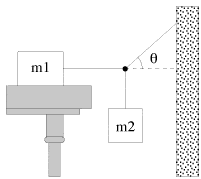# Need help with problem

## Homework Statement

Hi, I have a problem here and I need some help on it. The problem is this:block m$$_{1}$$ weighs 875 N. The coefficient of static friction between the block and the table is 0.24 and the angle theta is 25.0$$\circ$$. Find the maximum weight of block m$$_{2}$$ for which block m$$_{1}$$ will remain at rest.

2. The attempt at a solution

So far I've drawn an FBD for m$$_{1}$$, m$$_{2}$$, and the knot that connects them.

For the block m$$_{1}$$, I have T$$_{1}$$ = $$M$$$$_{s}$$N in the x-direction, and N = m$$_{1}$$g for the y-direction.

For block m$$_{2}$$, I have T$$_{2}$$ = m$$_{2}$$g in the y-direction.

For the knot, I have T$$_{1}$$ = T$$_{3}$$*cos(25) in the x-direction and T$$_{2}$$ = T$$_{3}$$*sin(25) in the y-direction.

Since T$$_{1}$$ = T$$_{3}$$*cos(25), and T$$_{1}$$ = $$M$$$$_{s}$$N, I set T$$_{3}$$*cos(25) = $$M$$$$_{s}$$N and solving for T$$_{3}$$, I get T$$_{3}$$ = ($$M$$$$_{s}$$N)/(cos(25))

In the knot, we have T$$_{2}$$ = T$$_{3}$$*sin(25). I plug in the T$$_{3}$$ derived earlier and T$$_{2}$$ = m$$_{2}$$g into this equation to get m$$_{2}$$g = $$M$$$$_{s}$$*m$$_{1}$$g*tan(25)

When I plug in numbers, the answer I get is about 98, and that just doesn't seem right to me. Did I do something wrong? Thanks for the help in advance.

learningphysics
Homework Helper
I get the same answer as you. 97.9N. Why do you think it is the wrong answer?

I get the same answer as you. 97.9N. Why do you think it is the wrong answer?

I've tried putting that in as the answer, but the computer says it isn't correct.

learningphysics
Homework Helper
I've tried putting that in as the answer, but the computer says it isn't correct.

Did you put in 98 or 97.9? Maybe they want the mass and not the weight? I'm not sure...

Did you put in 98 or 97.9? Maybe they want the mass and not the weight? I'm not sure...

When I put it in, it wants the units as newtons, so I'm sure it's weight. But now that I have a second opinion, I'll just argue with the professor if it's wrong =]. Thanks!

Did you neglect the static friction force?

which would be 210N.. So whatever Tension you assigned to the rope being pulled horizontally would be a maximum of 210N

Yes it seems you did cause I also get 97.9N as the answer....

If it helps I am also a Physics teacher for the sake of your arguement.

I did the problem, and I also got 97.9 N. Just to let you feel more secure about it :)

Alright! Thanks everyone!